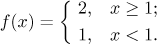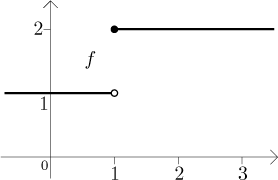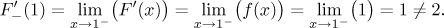Example: Consider the functionThis function looks like this:We claim that it has no antiderivative on [0,2]. We will prove it by contradiction.

Assume that some function F is an antiderivative of f on [0,2]. Then for x > 1 we have F ′(x) = f (x) = 2, while for x < 1 we have F ′(x) = f (x) = 1.

What can be said about the derivative at x = 1? Since F is an antiderivative, we must have F ′(1) = f (1) = 2. Therefore also the derivative of F at 1 from the left must exist and be equal to 2. However, since F is continuous at 1 from the left and F ′ is continuous on (0,1) (a left neighborhood of 1), we can use theorem (see Derivative and limit in Derivatives - Theory - MVT) to evaluate the left derivative at 1:This contradiction shows that our assumption was wrong, F cannot be an antiderivative of f.